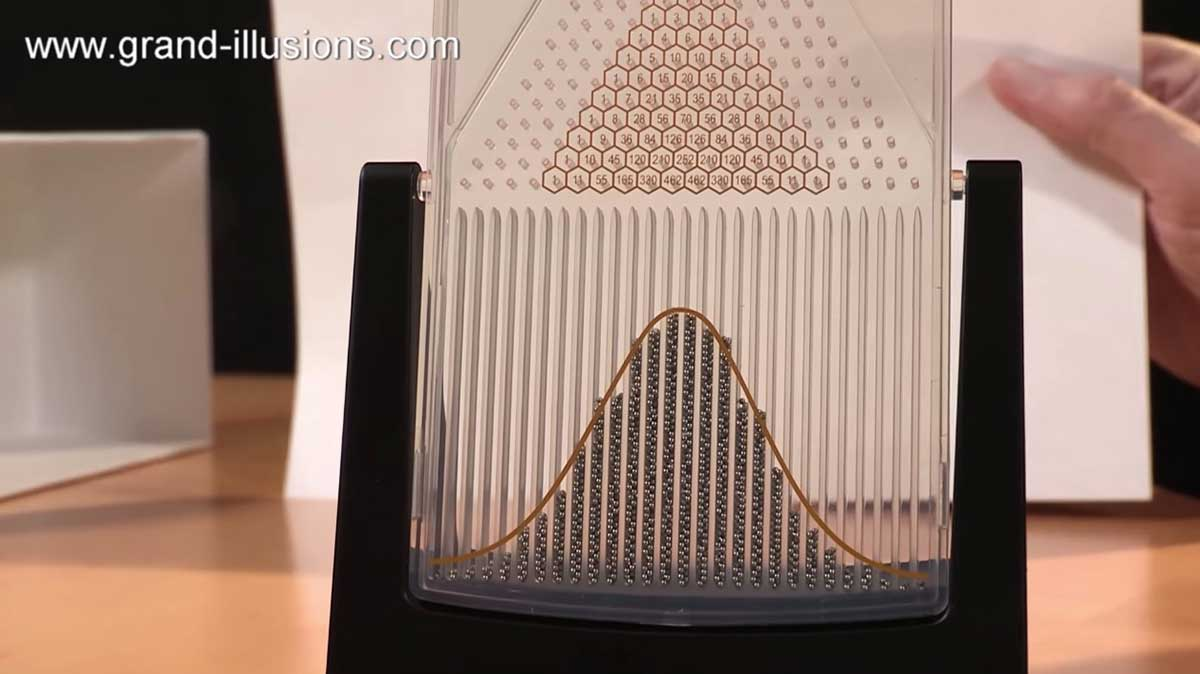## Desktop probability machineTim of Grand Illusions shows off this nifty normal curve distribution demonstration machine called the Galton Board. It has 3,000 tiny metal beads that fall through a grid of pegs, landing into one of 28 columns at the bottom. Each time a bead hits a peg, it moves to the left or the right. It's rare for a bead to fall to the left every time it hits a peg on the way down. It's equally rare for it to fall right every time it hits a peg on the way down. That's why most of the beads end up in the columns in the center.

The Galton Board is math in motion, demonstrating centuries-old mathematical concepts in an innovative desktop device. It incorporates Sir Francis Galton’s (1822-1911) illustration of the binomial distribution, which for a large number of beads approximates the normal distribution. It also has a superimposed Pascal’s Triangle (Blaise Pascal, 1623-1662), which is a triangle of numbers that follows the rule of adding the two numbers above to get the number below. The number at each peg represents the number of different paths a bead could travel from the top peg to that peg. The Fibonacci numbers (Leonardo Fibonacci, 1175-1250), can also be found as the sums of specific diagonals in the triangle. When rotated on its axis, the 3,000 beads cascade through rows of symmetrically placed pegs in the desktop-sized Galton Board. When the device is level, each bead bounces off the pegs with equal probability of moving to the left or right.

Read the rest